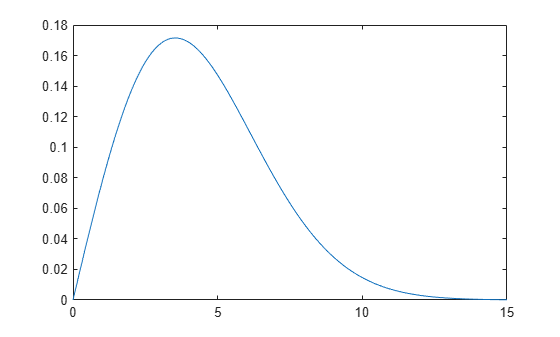Documentation

### This is machine translation

Mouseover text to see original. Click the button below to return to the English version of the page.

# median

Median of probability distribution

## Syntax

``m = median(pd)``

## Description

example

````m = median(pd)` returns the median `m` for the probability distribution `pd````

## Examples

collapse all

Load the sample data. Create a vector containing the first column of students' exam grade data.

```load examgrades x = grades(:,1);```

Create a normal distribution object by fitting it to the data.

`pd = fitdist(x,'Normal')`
```pd = NormalDistribution Normal distribution mu = 75.0083 [73.4321, 76.5846] sigma = 8.7202 [7.7391, 9.98843] ```

Compute the median of the fitted distribution.

`m = median(pd)`
```m = 75.0083 ```

For a symmetrical distribution such as the normal distribution, the median is equal to the mean, `mu`.

Create a Weibull probability distribution object.

`pd = makedist('Weibull','a',5,'b',2)`
```pd = WeibullDistribution Weibull distribution A = 5 B = 2 ```

Compute the median of the distribution.

`m = median(pd)`
```m = 4.1628 ```

For a skewed distribution such as the Weibull distribution, the median and the mean may not be equal.

Calculate the mean of the Weibull distribution and compare it to the median.

`mean = mean(pd)`
```mean = 4.4311 ```

The mean of the distribution is greater than the median.

Plot the pdf to visualize the distribution.

```x = [0:.1:15]; pdf = pdf(pd,x); plot(x,pdf)```## Input Arguments

collapse all

Probability distribution, specified as a probability distribution object created using one of the following.

Function or AppDescription
`makedist`Create a probability distribution object using specified parameter values.
`fitdist`Fit a probability distribution object to sample data.
Distribution FitterFit a probability distribution to sample data using the interactive Distribution Fitter app and export the fitted object to the workspace.

## Output Arguments

collapse all

Median of the probability distribution, returned as a scalar value. The value of `m` is the 50th percentile of the probability distribution.